# How to Calculate and Solve for Universal Soil Loss | Water Budget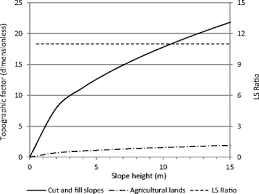The image above represents universal soil loss.

To compute for universal soil loss, six essential parameters are needed and these parameters are Rainfall and Run off Activity Index by Geological Location (R), Soil Erodibility Factor (K), Topographic Factor (LS), Yield Slope (S), Cropping Management Factor (C) and Conservation Practice Factor (P).

The formula for calculating universal soil loss:

A = 2.24(R)(K) . (LS) . (C)(P)

Where:

A = Universal Soil Loss
R = Rainfall and Run off Activity Index by Geological Location
K = Soil Erodibility Factor
LS = Topographic Factor
S = Yield Slope
C = Cropping Management Factor
P = Conservation Practice Factor

Let’s solve an example;
Find the universal soil loss when the rainfall and run off activity index by geological location is 12, the soil erodibility factor is 8, the topographic factor is 9, yield scope is 4, cropping management factor is 2 and the conservation practice factor is 7.

This implies that;

R = Rainfall and Run off Activity Index by Geological Location = 12
K = Soil Erodibility Factor = 8
LS = Topographic Factor = 9
S = Yield Slope = 4
C = Cropping Management Factor = 2
P = Conservation Practice Factor = 7

A = 2.24(R)(K) . (LS) . (C)(P)
A = 2.24(12)(8) . (9) . (2)(7)
A = 2.24(12096)
A = 27095.04

Therefore, the universal soil loss is 27095.04.

Calculating for Rainfall and Run off Activity Index by Geological Location when the Universal Soil Loss, the Soil Erodibility Factor, the Topographic Factor, the Cropping Management Factor and the Conservation Practice Factor is Given.

R = A / 2.24(K) (LS) C.P

Where:

R = Rainfall and Run off Activity Index by Geological Location
A = Universal Soil Loss
K = Soil Erodibility Factor
LS = Topographic Factor
C = Cropping Management Factor
P = Conservation Practice Factor

Let’s solve an example;
Find the rainfall and run off activity index by geological location when the universal soil loss is 12, the soil erodibility factor is 8, the topographic factor is 10, the cropping management factor is 4 and the conservation practice factor is 7.

This implies that;

A = Universal Soil Loss = 12
K = Soil Erodibility Factor = 8
LS = Topographic Factor = 10
C = Cropping Management Factor = 4
P = Conservation Practice Factor = 7

R = A / 2.24(K) (LS) C.P
R = 12 / 2.24(8) (10) (4)(7)
R = 12 / 2.24(8) (10) (4)(7)
R = 12 / 2.24(2240)
R = 12 / 5017.6
R = 0.00239

Therefore, the Rainfall and run off activity index by geological location is 0.00239.

Calculating for Soil Erodibility Factor when the Universal Soil Loss, the Rainfall and Run off Activity Index by Geological Location, the Topographic Factor, the Cropping Management Factor and the Conservation Practice Factor is Given.

K = A / 2.24(R) (LS) C.P

Where:

K = Soil Erodibility Factor
R = Rainfall and Run off Activity Index by Geological Location
A = Universal Soil Loss
LS = Topographic Factor
C = Cropping Management Factor
P = Conservation Practice Factor

Let’s solve an example;
Find the soil erodibility factor when the rainfall and run off activity index by geological location is 20, the universal soil loss is 15, the topographic factor is 2, the cropping management factor is 9 and the conservation practice factor is 6.

This implies that;

R = Rainfall and Run off Activity Index by Geological Location = 20
A = Universal Soil Loss = 15
LS = Topographic Factor = 2
C = Cropping Management Factor = 9
P = Conservation Practice Factor = 6

K = A / 2.24(R) (LS) C.P
K = 15 / 2.24(20) (2) (9)(6)
K = 15 / 4838.4
K = 0.0031

Therefore, the soil erodibility factor is 0.0031.

Calculating for the Topographic Factor when the Universal Soil Loss, the Rainfall and Run off Activity Index by Geological Location, the Soil Erodibility Factor, the Cropping Management Factor and the Conservation Practice Factor is Given.

LS = A / 2.24(R)(K) C.P

Where:

LS = Topographic Factor
R = Rainfall and Run off Activity Index by Geological Location
A = Universal Soil Loss
K = Soil Erodibility Factor
C = Cropping Management Factor
P = Conservation Practice Factor

Let’s solve an example;
Find the topographic factor when the rainfall and run off activity index by geological location is 14, the universal soil loss is 32, the soil erodibility factor is 8, the cropping management factor is 20 and the conservation practice factor is 2.

This implies that;

R = Rainfall and Run off Activity Index by Geological Location = 14
A = Universal Soil Loss = 32
K = Soil Erodibility Factor = 8
C = Cropping Management Factor = 20
P = Conservation Practice Factor = 2

LS = A / 2.24(R)(K) C.P
LS = 32 / 2.24(14)(8) (20)(2)
LS = 32 / 2.24(4480)
LS = 32 / 10035.2
LS = 0.003188

Therefore, the topographic factor is 0.003188.

Calculating for the Cropping Management Factor when the Universal Soil Loss, the Rainfall and Run off Activity Index by Geological Location, the Soil Erodibility Factor, the Topographic Factor and the Conservation Practice Factor is Given.

C = A / 2.24(R)(K) (LS) P

Where:

C = Cropping Management Factor
R = Rainfall and Run off Activity Index by Geological Location
A = Universal Soil Loss
K = Soil Erodibility Factor
LS = Topographic Factor
P = Conservation Practice Factor

Let’s solve an example;
Find the cropping management factor when the rainfall and run off activity index by geological location is 3, the universal soil loss is 40, the soil erodibility factor is 1, the topographic factor is 7 and the conservation practice factor is 10.

This implies that;

R = Rainfall and Run off Activity Index by Geological Location = 3
A = Universal Soil Loss = 40
K = Soil Erodibility Factor = 1
LS = Topographic Factor = 7
P = Conservation Practice Factor = 10

C = A / 2.24(R)(K) (LS) P
C = 40 / 2.24(3)(1) (7) 10
C = 40 / 2.24(210)
C = 40 / 470.4
C = 0.085

Therefore, the cropping management factor is 0.085.

Calculating for the Conservation Practice Factor when the Universal Soil Loss, the Rainfall and Run off Activity Index by Geological Location, the Soil Erodibility Factor, the Topographic Factor, Yield Scope and the Cropping Management Factor is Given.

P = A / 2.24(R)(K) (LS) C

Where:

P = Conservation Practice Factor
R = Rainfall and Run off Activity Index by Geological Location
A = Universal Soil Loss
K = Soil Erodibility Factor
LS = Topographic Factor
C = Cropping Management Factor

Let’s solve an example;
Find the conservation practice factor when the rainfall and run off activity index by geological location is 10, the universal soil loss is 5, the soil erodibility factor is 8, the topographic factor is 2 and the cropping management factor is 11.

This implies that;

R = Rainfall and Run off Activity Index by Geological Location = 10
A = Universal Soil Loss = 5
K = Soil Erodibility Factor = 8
LS = Topographic Factor = 2
C = Cropping Management Factor = 11

P = A / 2.24(R)(K) (LS) C
P = 5 / 2.24(10)(8) (2) 11
P = 5 / 2.24(1760)
P = 5 / 3942.4
P = 0.00126

Therefore, the conservation practice factor is 0.00126.

Nickzom Calculator – The Calculator Encyclopedia is capable of calculating the universal soil loss.

To get the answer and workings of the universal soil loss using the Nickzom Calculator – The Calculator Encyclopedia. First, you need to obtain the app.

You can get this app via any of these means:

To get access to the professional version via web, you need to register and subscribe for NGN 1,500 per annum to have utter access to all functionalities.
You can also try the demo version via https://www.nickzom.org/calculator

Apple (Paid) – https://itunes.apple.com/us/app/nickzom-calculator/id1331162702?mt=8
Once, you have obtained the calculator encyclopedia app, proceed to the Calculator Map, then click on Agricultural under Engineering.Now, Click on Water Budget under Agricultural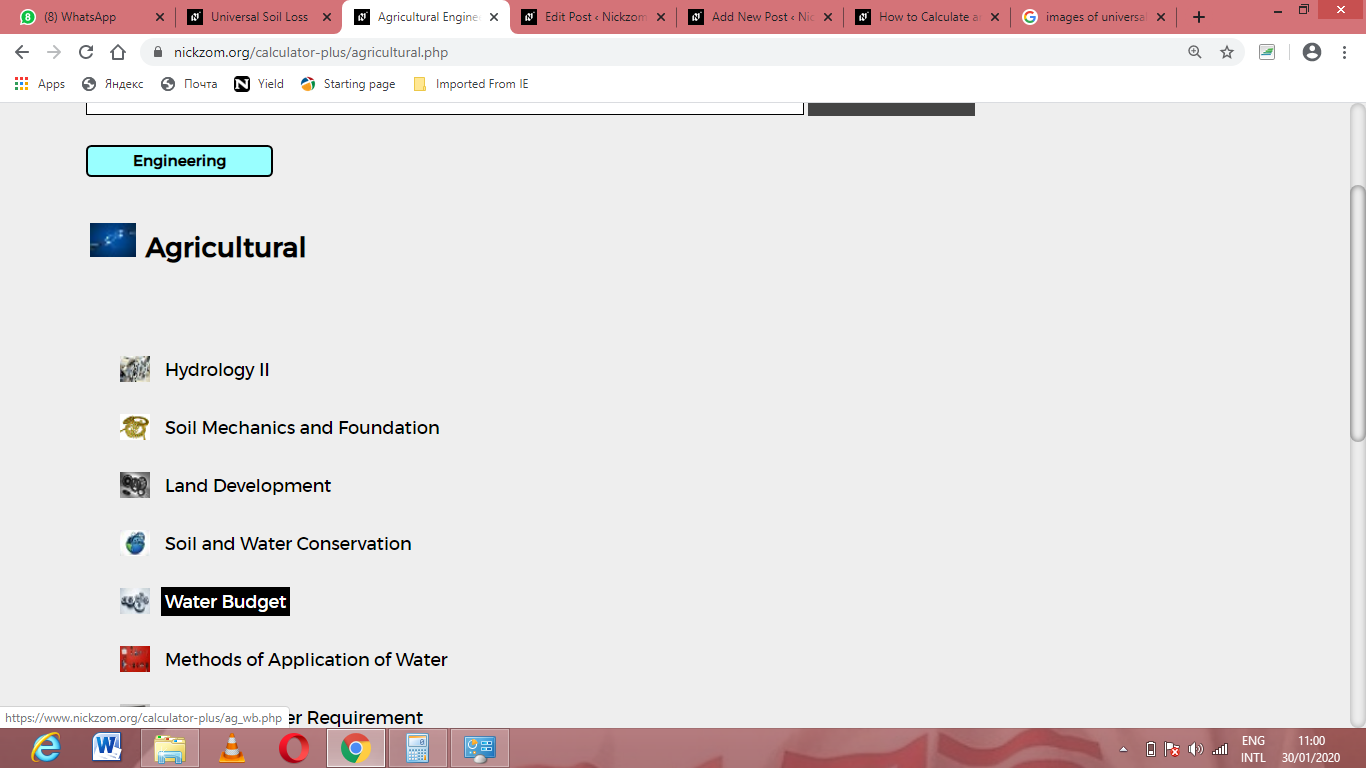Now, Click on Universal Soil Loss under Water Budget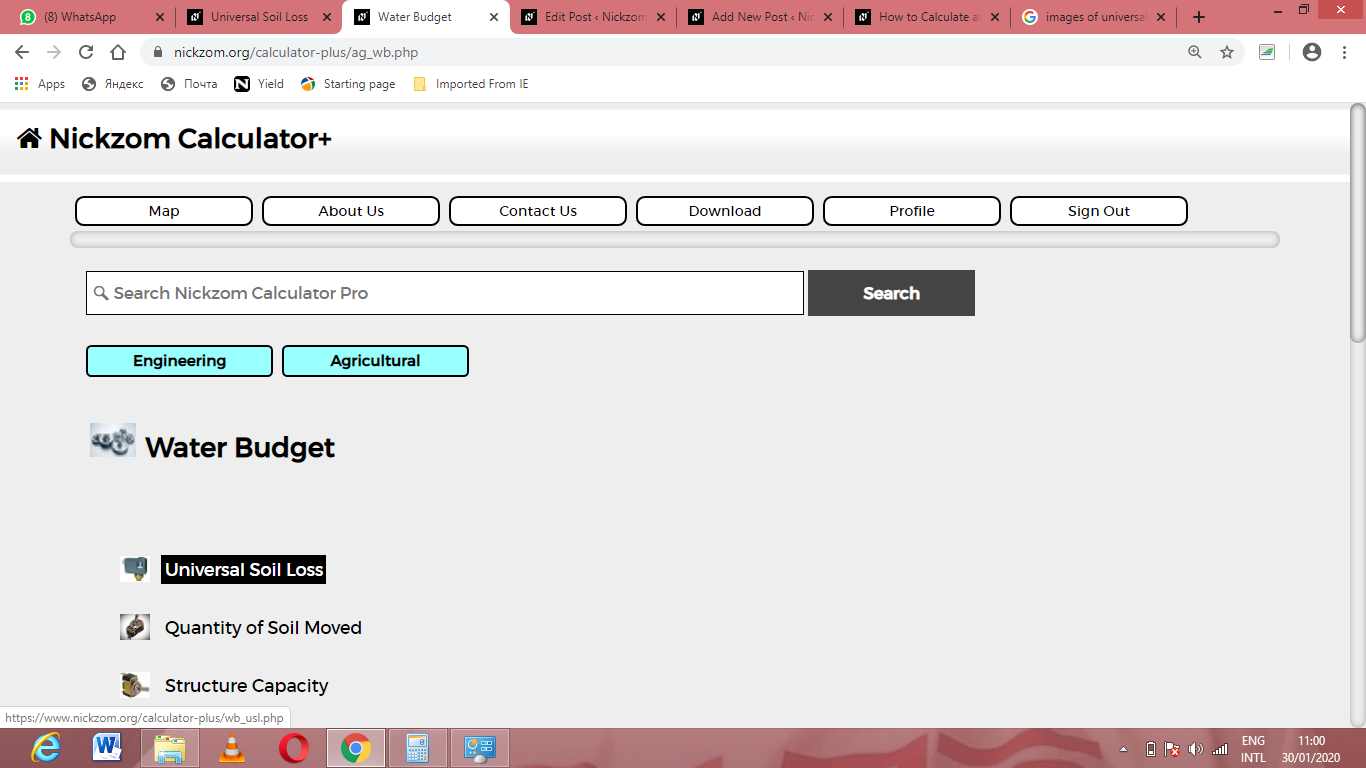The screenshot below displays the page or activity to enter your values, to get the answer for the universal soil loss according to the respective parameters which are the Rainfall and Run off Activity Index by Geological Location (R), Soil Erodibility Factor (K), Topographic Factor (LS), Yield Slope (S), Cropping Management Factor (C) and Conservation Practice Factor (P).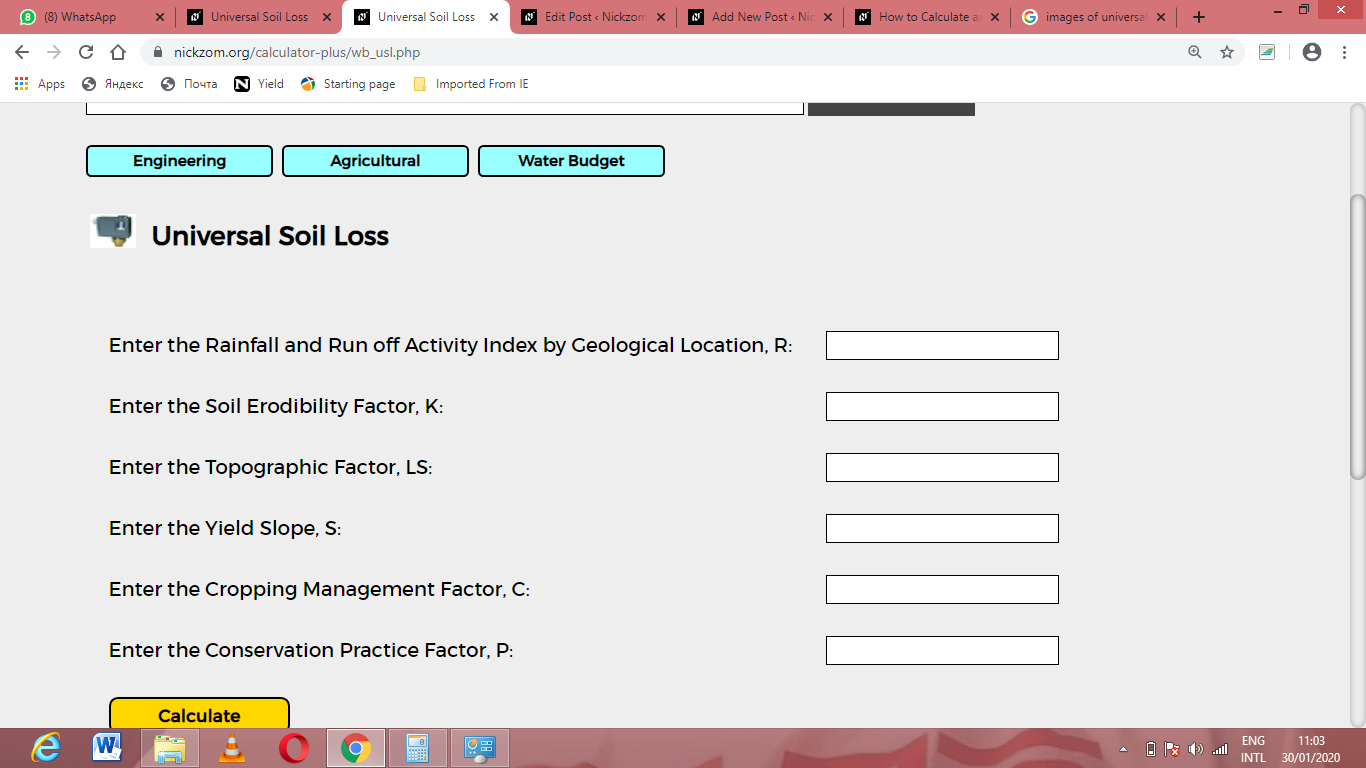Now, enter the values appropriately and accordingly for the parameters as required by the Rainfall and Run off Activity Index by Geological Location (R) is 12, Soil Erodibility Factor (K) is 8, Topographic Factor (LS) is 9, Yield Slope (S) is 4, Cropping Management Factor (C) is 2 and Conservation Practice Factor (P) is 7.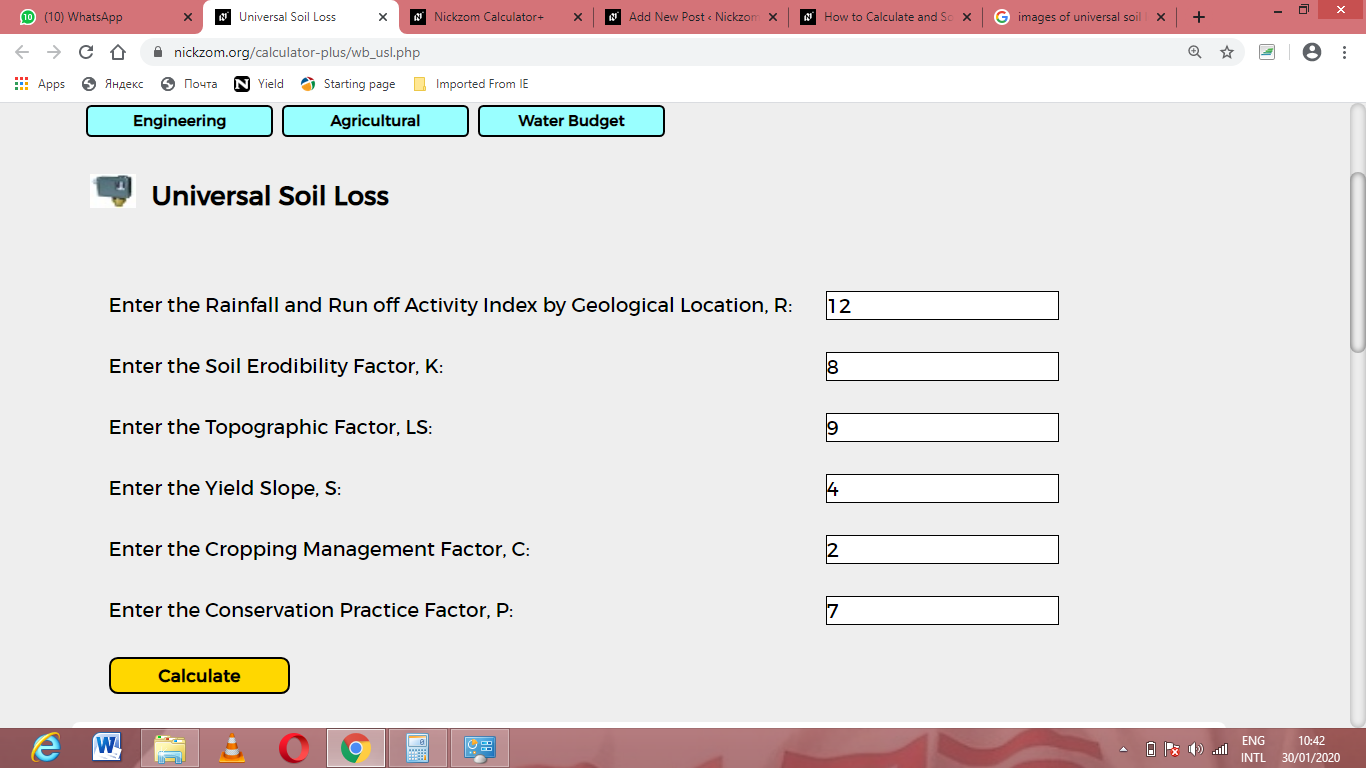Finally, Click on Calculate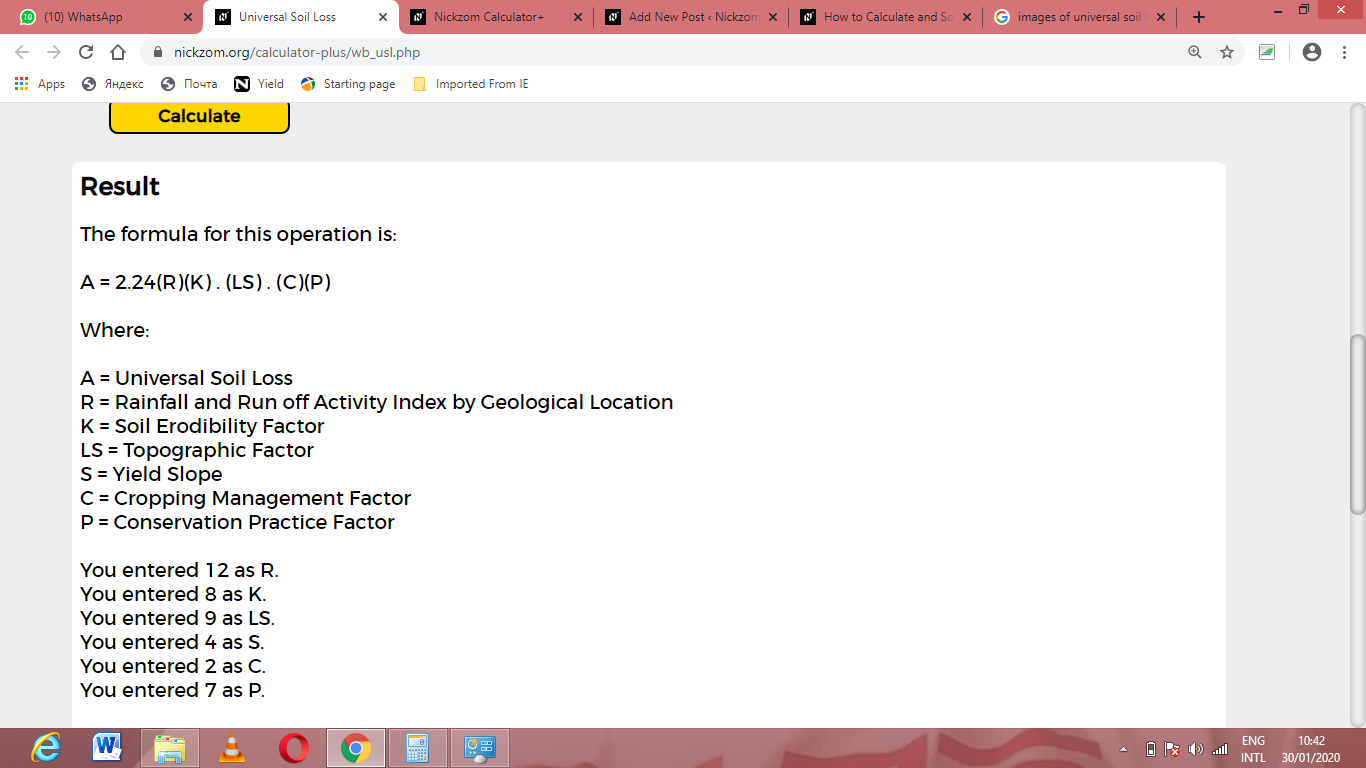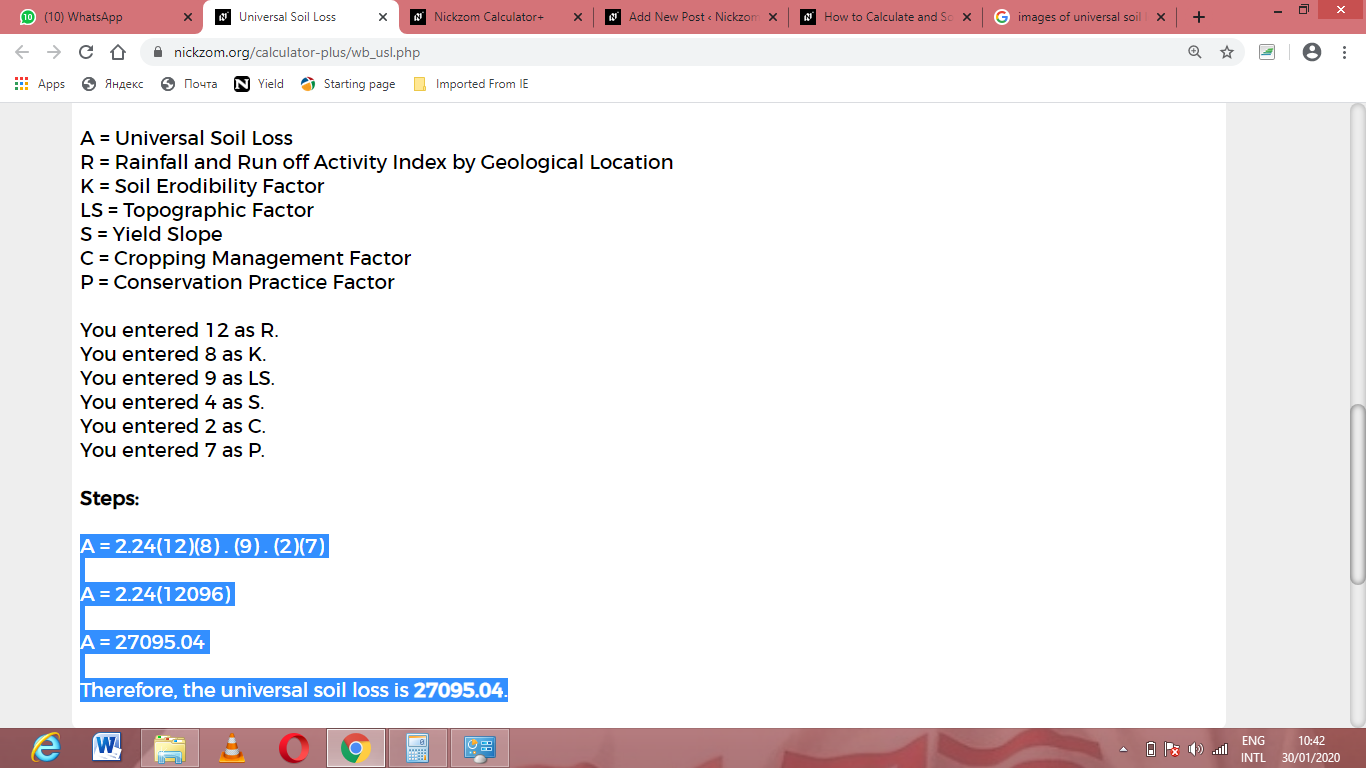As you can see from the screenshot above, Nickzom Calculator– The Calculator Encyclopedia solves for the universal soil loss and presents the formula, workings and steps too.